International Journal of Geomagnetism and Aeronomy
Vol 1, No. 3, August 1999

# Magnetic anomaly field of the Earth from gradient measurements at stratospheric altitudes

Yu. P. Tsvetkov, N. M. Rotanova, V. N. Oraevskiy, A. L. Kharitonov, and S. D. Odintsov

Institute of Terrestrial Magnetism, Ionosphere, and Radio Wave Propagation, Troitsk, Moscow Region, Russia

### Abstract

Data on the magnetic anomaly field (MAF) obtained from gradient measurements by stratospheric balloons and the MAGSAT satellite are analyzed. The methods used for deriving these fields are described, and the consistency between the two data sets is demonstrated. The data on MAF and its vertical gradient have been used to construct a lithospheric geophysical cross section that characterizes the deposition depths of MAF sources. A detailed analysis of the balloon flight for j = 55 o N and l = 81o - 121 o E was performed; some other geophysical parameters were invoked for it. By using the MAF data for different altitudes and by solving the direct problem, it is shown that the anomalies observed in the cross section are fairly well described by a number of isolated sources whose magnetizations and depths do not contradict current ideas about the magnetic field of the Earth's crust.

### Introduction

The main goals of the investigation of the magnetic anomaly field (MAF) and magnetically active lithospheric sources are directly associated with research into the interpretation of the regional and long-wave components of the anomalous field. Here we encounter the problem of separating different components from the near-Earth measurements [Pashkevich et al., 1990]. However, at distances from the surface of the Earth comparable with the vertical thickness of the magnetically active lithospheric layer, the effects of the surface and deep sources become almost equal, and a smooth field originating from sources located throughout the layer is observed. For instance, at stratospheric heights of ~30 km, the MAF is represented by magnetic anomalies ranging from regional anomalies with a transverse size of ~30 km up to those with the longest wavelengths. If we consider the MAF as a whole, magnetic measurements at stratospheric altitudes successfully supplement aeromagnetic and satellite surveys (see, e.g., Achache et al. ).

This paper analyzes the results of magnetic gradient measurements at stratospheric altitudes and the MAGSAT satellite data. The results of processing these two experiments are used to determine the sources of regional magnetic anomalies.

### Observational Data and Their Processing

Three transcontinental balloon flights at heights of H = 303 km along latitudinal routes up to 5000-8000 km in length have been performed over Russia [Tsvetkov, 1993]. Each of the balloons was equipped with two proton magnetometers whose detectors were separated by a vertical distance of 2.5 km. The magnetometers operated in a cyclic mode with one measurement per minute at the average flight velocity of 40-50 km h -1. The magnetic field was measured synchronously by the two magnetometers, and hence the normalized differences between these measurements were taken to be the vertical gradient of the magnetic field. The measurement baseline (the distance between the magnetic field detectors) was ~1/20 of the distance to the sources, and hence the data obtained can only be regarded approximately as gradient data. In fact, the measurements are values of the vertical gradient at stratospheric heights averaged over a distance of 2.5 km.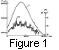The measurements of the intensity of the vector field modulus T and its vertical gradient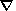T for one of the balloon flights in a westward direction from Kamchatka along the j = 55 o Nparallel are shown in Figure 1. Figure 2 shows the MAF parameters obtained from that stratospheric balloon flight.

During the data processing, the normal field represented by an analytical model and corrected for secular variation was subtracted from the measured field. The resulting differences were taken to be the anomalous field. An analogous procedure was used to obtain the vertical gradient of the magnetic field. The measuring errors have been considered by Tsvetkov , where the standard deviation of the MAF gradient measurements was estimated to be 0.3 nT km -1, with the major contribution coming from the operating conditions of the detector on a moving platform. The standard deviation of the MAF vertical gradient along the whole flight length from Kamchatka to the Urals was s(DT)a30 = 2.2  nT km -1, while for the MAF itself s (DT)a30 = 45 nT. The root-mean-square value of the gradient for flight path segments 600 km in length (Figure 2a) was estimated to be in the range from 0.3 nT km -1 (eastern and central parts of the Sea of Okhotsk) to 3 nT km -1 (the Baikal fold system).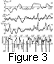The long-wave components of the MAF spectrum are also clearly observed at satellite heights. The best observational information for the study of the anomalous field from space is the MAGSAT satellite data [Cohen et al., 1986; Langel et al., 1982]. The field intensity at satellite heights ranges from 0 nT to 30 nT. During the satellite data processing, only the field values at positions corresponding to the above mentioned balloon flight route along the j = 55 o N parallel were chosen in order to find the anomalous fields and compare them with the gradient data for stratospheric heights. Then the data were processed to eliminate random outliers and filtered with the critical period of ~16 points to exclude the diurnal periodicity. From the filtered data, only those from intervals for which the geomagnetic activity index of Kp2 were selected. Figure 3 shows the anomalous magnetic field for the balloon flight route between l = 81 o E and l = 122 o E, where Figure 3a illustrates the MAF at a height of 350 km (from the MAGSAT data), and Figures 3b and 3c show the MAF and its vertical gradient for a height of 30 km, respectively (the balloon data).

Attention is drawn to the fairly good agreement between anomalies at satellite and balloon altitudes, though some differences are observed. This is due to the fact that the anomalous field at the measurement point is a superposition of the fields of sources which are mainly in the spherical segment whose intersection with the Earth's surface is limited by a circle with a radius nearly equal to the magnetic survey height [Lugovenko et al., 1990]. Therefore the anomalous field at the measurement point at satellite altitudes is formed by the superposition of the fields from sources within an area approximately 100 times larger than that for a height of ~30 km. This can explain some of the differences between the curves shown in Figures 3a, 3b, and 3c.

### Results

To interpret geophysically the results shown in Figures 3a, 3b, and 3c, a vertical geophysical lithospheric cross section was first obtained. To this end, the expression determining the MAF source depths derived from the empirical decrease in magnetic anomaly field with measurement height was used [Tsvetkov et al., 1995, 1997]: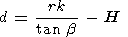where d is the source depth, r is the correlation radius of the autocorrelation function, b is the angle of inclination of the tangent to the curve of anomalous field amplitude versus height of measurements, and k is a proportionality factor.

Figure 3d shows the variations in this depth along the route considered. It also gives information on some auxiliary geophysical parameters. The question arises as to whether the constructed cross section is reliable and consistent with other data. To this end, nearly all the available geophysical information for the region under study was used, and a complex analysis was performed. It showed that the horizontal boundaries of the layers are also the boundaries of the extent of abyssal breaks in the Earth's crust (which is shown in Figure 3d for the Mohorovichich boundary), the vertical discontinuities of the horizontal layers being more pronounced in the magnetic data. This allows us to divide the section into blocks in both the horizontal and vertical directions, according to geological properties.

For studies of the geomagnetic field along the balloon route, it is also important to know whether the rock magnetization responsible for the magnetic anomalies in the region of interest has an inductive or a remanent character. It is known that the net magnetization of rocks is given by

JZs= JZr+ JZi

JHs= JHr+ JHi

where Jr and Ji characterize the remanent (Jr) and inductive (Ji) magnetizations.

By assuming that MAF is fully associated with the magnetization of the lithosphere, we can writewhere Za is a vertical component of MAF, and Ha is a horizontal component of MAF, or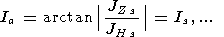Thus if the anomalous inclinations ( Ia ) calculated from the above expressions differ in sign from the inclination of the current main magnetic field of the Earth which varies appreciably in time and space, we can assume a predominantly remanent magnetization. Using the above equations, the directions of the net magnetization vectors along the balloon flight route were calculated from the MAGSAT data. It turned out that for the region of the Baikal anomaly of electric conductivity [Rokityanskiy, 1975] which is located between l = 102 o E and l = 113 o E, the inclination Is coincides in sign with the direction of the main magnetic field vector. This indicates that inductive magnetization dominates in this region. As to the remaining part of the profile, the values of Is are opposite in sign to the direction of the main field vector inclination. This suggests that remanent magnetization dominates.

Using observational data for different heights and techniques for solving the direct problem, the depths of the magnetic sources were determined. The relatively simple shape of the MAF curves for stratospheric altitudes allows us to find the strangest anomalies and interpret them one at a time. To minimize the non-uniqueness of the set of models for the field sources, the magnetic field distribution for individual sources was found, and a wide range of initial parameters (source depth, magnetic moment) was used to eliminate the discrepancy between the calculated field and the field measured along the flight route. As the field model, the analytical expression for the two-dimensional problem [Kolyubakin and Lapina, 1960]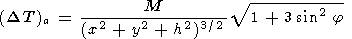was used. Here M is the magnetic moment of an individual source, x and y are the Cartesian coordinates with respect to an origin at distance h above the source, and j is the magnetic latitude. According to the superposition concept, horizontal magnetized strata and horizontal and vertical prismatic bodies were represented by different combinations of different sources. To limit the number of choices, the individual sources were correlated with the geometries of anomalies at the Earth's surface. The direct problem was solved separately for anomalies 1-4 shown in Figure 3b and for the low-frequency trend of 1, 2 and 3, 4.

The parameters defining the theoretical magnetic field were the depths of the sources, their magnetic moments associated with the rock magnetization intensity, and the geometrical distribution of individual sources corresponding to the anomalous magnetization area. The choice was considered to be optional when the theoretical and observed parameters, that is, the intensities and vertical gradients of the magnetic field anomalies and the extent of the anomalies at the level of half the maximum intensity of the anomaly, coincided simultaneously. It turned out that in choosing the optimum models, the discrepancy between the model and measured fields could be successfully corrected by the function of the MAF vertical gradient which points to its high significance.

The shapes of the magnetic source bodies were determined from the spatial distribution of such simulating sources. The vertical sizes of these sources were estimated from the magnetization of rocks typical of this region. In other words, the evaluation of the source vertical thickness required to create the calculated magnetic moment was carried out. The magnetic anomalies shown in Figure 3 have sources in the form of horizontal plates (strata) with a minimum width of ~20 km and a thickness of several kilometers. The characteristic depths of the magnetic centers of these sources are 5, 15-20, and 30-35 km, which does not contradict current ideas about anomalous field sources. Thus our analysis of the MAF stratospheric observations shows that the sources of the regional magnetic anomalies can occur at any depth limited by the upper and lower edges of the magnetoactive lithospheric layer. Consequently, the classification of the anomalies in terms of source depths is not physically justified.

### Conclusions

1. Profiles of the anomalous magnetic field and its vertical gradient have been obtained by processing the stratospheric geomagnetic measurements and MAGSAT satellite data for the flight route along the j = 55 o N parallel. The analysis and interpretation of the profiles have revealed the significance of stratospheric gradient measurements for solving fundamental problems for the Earth's crustal magnetic field.

2. Using all the available geophysical data and taking into account the calculations of the anomalous inclination, it has been found that inductive magnetization dominates in some regions (for instance, in the region between l = 102 o E and l = 113 o E) along with remanent magnetization as a basic source of the anomalous field.

3. Using the anomalous field data for different heights, the vertical geophysical cross section of the lithosphere has been constructed. The boundaries of the horizontal lithospheric layers coincide with the boundaries of the extent of abyssal breaks.

4. Solution of the direct problem has shown that the regional magnetic anomaly sources can occur at any depth limited by the upper and lower edges of the lithospheric magnetoactive layer. In this case the depth of the latter does not contradict current ideas about anomalous field sources.

#### Acknowledgments

This work was supported by the Russian Foundation for Basic Research (projects 94-05-16346 and 94-05-17517).

### References

Achache, J., Y. Cohen, and G. Unal, The French program of circumterrestrial magnetic surveys using stratospheric balloons, EOS Trans. AGU, 72, 100-101, 1991.

Cohen, Y., M. Menvielle, and J.-L. Le Mouël, Magnetic measurements aboard a stratospheric balloon, Phys. Earth Planet Inter., 44, 348-357, 1986.

Kolyubakin, V. V., and M. I. Lapina, A Review of Methods for Solution of the Direct and Inverse Problems of Magnetic Prospecting, in Trudy IFZ AN SSSR, no. 13 (180), 363 pp., Akad. Nauk SSSR, Moscow, 1960.

Langel, R. A., C. C. Schnetzler, J. D. Phillips, and R. J. Horner, Initial vector magnetic anomaly map from MAGSAT, Geophys. Res. Lett., 9 (4), 273-276, 1982.

Lugovenko, V. N., et al., Statistical characteristics of anomalous geophysical fields and their relation with the structure of the Earth's crust, preprint 12 (897), 26 pp., IZMIRAN, Moscow, 1990.

Pashkevich, I. K., et al., Magnetic Model of the Lithosphere of Europe, 165 pp., Naukova Dumka, Kiev, 1990.

Rokityanskiy, I. I., Investigation of Anomalies of Electrical Conductivity Using the Technique of Magneto-Variational Contouring, 279 pp., Naukova Dumka, Kiev, 1975.

Tsvetkov, Yu. P., Investigation of the anomalous magnetic field of the Earth at stratospheric altitudes, Geomagn. Aeron., 33, 159-164, 1993.

Tsvetkov, Yu. P., V. A. Belkin, Kh. D. Kanonidi, and A. L. Kharitonov, Physical-geological interpretation of the anomalous magnetic field measured in the stratosphere, Fiz. Zemli, 35 (4), 54-57, 1995.

Tsvetkov, Yu. P., N. M. Rotanova, V. N. Oraevsky, and S. D. Odintsov, Magnetic anomaly fields determined from gradient measurement at stratospheric altitudes and from MAGSAT satellite data, Geomagn. Geoelectr., 49, 689-699, 1997.

Load files for printing and local use. This document was generated by TeXWeb (Win32, v.2.0) on September 12, 1999.